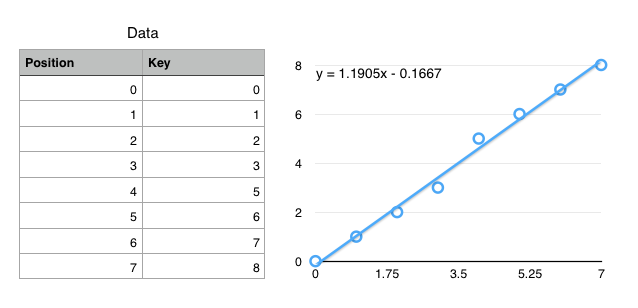CSE-4/562 Spring 2021 - Indexing (Part 2) and Views

March 4, 2021

### Today

1. How do we keep a list sorted under updates?
2. Can we "compress" an ISAM index?
3. Other ways to accelerate queries.

### Access Paths

Reads Want: Nice sorted, compact list.

Writes Want: Random order.

What happens if we optimize for reads
(and even do away with pages)

Each insert requires $O(N)$ write IOs.

"Write Amplification"

How can we reduce Write Amplification?

Idea: Buffer writes

Pro: With a $\mathcal B$ element buffer, $O(\frac{N}{\mathcal B})$ write amplification (amortized)

Con: Every read now needs to go to two places

Con: $O(\frac{N}{\mathcal B})$ is still linear

Idea: Don't merge!

Pro: No write amplification!

Con: Every read now needs to go to $O(\frac{N}{B})$ places on disk (Read Amplification)

Idea: Combine the two?

## Log-Structured Merge (LSM) Trees

• Buffer contains $\mathcal B$ records
• Level 1 contains $\mathcal B$ records
• Level 2 contains $2\mathcal B$ records
• Level 3 contains $4\mathcal B$ records

In general, level $i$ contains $2^{i-1} \mathcal B$ records.

When writing to a full level, merge and write to next level instead.

### Basic LSM Trees

Write Amplification: Every record copied $O(\log N)$ times

Read Amplification: At most $O(\log N)$ levels

### LSM Trees in Practice

• LevelDB/RocksDB
• Apache Cassandra
• HBase

### Other design choices

Fanout
Instead of doubling the size of each level, have each level grow by a factor of $K$. Level $i$ is merged into level $i+1$ when its size grows above $K^{i-1}$.
Store each level as $K$ sorted runs instead of proactively merging them. Merge the runs together when escalating them to the next level.

### CDF-Based Indexing

"The Case for Learned Index Structures"
by Kraska, Beutel, Chi, Dean, Polyzotis

### Cumulative Distribution Function (CDF)$f(key) \mapsto position$

(not exactly true, but close enough for today)

### Using CDFs to find records

Ideal: $f(k) = position$
$f$ encodes the exact location of a record
Ok: $f(k) \approx position$
$\left|f(k) - position\right| < \epsilon$
$f$ gets you to within $\epsilon$ of the key
Only need local search on one (or so) leaf pages.

Simplified Use Case: Static data with "infinite" prep time.

### How to define $f$?

• Linear ($f(k) = a\cdot k + b$)
• Polynomial ($f(k) = a\cdot k + b \cdot k^2 + \ldots$)
• Neural Network ($f(k) =$)

We have infinite prep time, so fit a (tiny) neural network to the CDF.

### Neural Networks

Extremely Generalized Regression
Essentially a really really really complex, fittable function with a lot of parameters.
Captures Nonlinearities
Most regressions can't handle discontinuous functions, which many key spaces have.
No Branching
if statements are really expensive on modern processors.
(Compare to B+Trees with $\log_2 N$ if statements)

### Summary

Tree Indexes
$O(\log N)$ access, supports range queries, easy size changes.
Hash Indexes
$O(1)$ access, doesn't change size efficiently, only equality tests.
LSM Trees
$O(K\log(\frac{N}{B}))$ access. Good for update-unfriendly filesystems.
CDF Indexes
$O(1)$ access, supports range queries, static data only.

## Views


SELECT partkey
FROM lineitem l, orders o
WHERE l.orderkey = o.orderkey
AND o.orderdate >= DATE(NOW() - '1 Month')
ORDER BY shipdate DESC LIMIT 10;


SELECT suppkey, COUNT(*)
FROM lineitem l, orders o
WHERE l.orderkey = o.orderkey
AND o.orderdate >= DATE(NOW() - '1 Month')
GROUP BY suppkey;


SELECT partkey, COUNT(*)
FROM lineitem l, orders o
WHERE l.orderkey = o.orderkey
AND o.orderdate > DATE(NOW() - '1 Month')
GROUP BY partkey;


All of these views share the same business logic!

Started as a convenience


CREATE VIEW salesSinceLastMonth AS
SELECT l.*
FROM lineitem l, orders o
WHERE l.orderkey = o.orderkey
AND o.orderdate > DATE(NOW() - '1 Month')


SELECT partkey FROM salesSinceLastMonth
ORDER BY shipdate DESC LIMIT 10;


SELECT suppkey, COUNT(*)
FROM salesSinceLastMonth
GROUP BY suppkey;


SELECT partkey, COUNT(*)
FROM salesSinceLastMonth
GROUP BY partkey;


But also useful for performance


CREATE MATERIALIZED VIEW salesSinceLastMonth AS
SELECT l.*
FROM lineitem l, orders o
WHERE l.orderkey = o.orderkey
AND o.orderdate > DATE(NOW() - '1 Month')


Materializing the view, or pre-computing and saving the view lets us answer all of the queries on the view faster!

What if the query doesn't use the view?


SELECT l.partkey
FROM lineitem l, orders o
WHERE l.orderkey = o.orderkey
AND o.orderdate > DATE(’2015-03-31’)
ORDER BY l.shipdate DESC
LIMIT 10;


Can we detect that a query could be answered with a view?

(sometimes)

View Query User Query SELECT $L_v$ FROM $R_v$ WHERE $C_v$ SELECT $L_q$ FROM $R_q$ WHERE $C_q$

When are we allowed to rewrite this table?

View Query User Query SELECT $L_v$ FROM $R_v$ WHERE $C_v$ SELECT $L_q$ FROM $R_q$ WHERE $C_q$
$R_V \subseteq R_Q$
All relations in the view are part of the query join
$C_Q = C_V \wedge C'$
The view condition is 'weaker' than the query condition
$attrs(C') \cap attrs(R_V) \subseteq L_V$     $L_Q \cap attrs(R_V) \subseteq L_V$
The view doesn't project away needed attributes
View Query User Query SELECT $L_v$ FROM $R_v$ WHERE $C_v$ SELECT $L_q$ FROM $R_q$ WHERE $C_q$
SELECT $L_Q$
FROM $(R_Q - R_V)$, view
WHERE $C_Q$

## Summary

• For each relation, identify candidate indexes
• For each join, identify candidate indexes
• Identify candidate views
• Identify available join, aggregate, sort algorithms

Enumerate all possible plans

... then how do you pick? (more soon)